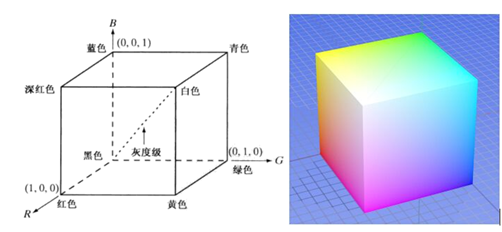• ## RGB转HSV

千次阅读 2016-11-27 22:57:27
RGB转HSV
基础知识介绍
RGB转为HSV后，可以更好的对颜色进行提取。应用于根据颜色对物体分类等场景。
RGB
大家都很熟悉，是红，绿，蓝三色的值。取值均为[0，255]  手机或者摄像机拍到的照片一般都是RGB24（深度是24bit，R、G、B分别占8位）或者RGB32。
HSV
HSV(Hue, Saturation, Value)是根据颜色的直观特性由A. R. Smith在1978年创建的一种颜色空间, 也称六角锥体模型(Hexcone Model)。 这个模型中颜色的参数分别是：色调（H），饱和度（S），亮度（V）。HSV颜色空间模型 色调H：用角度度量，取值范围为0°～360°，从红色开始按逆时针方向计算，红色为0°，绿色为120°,蓝色为240°。它们的补色是：黄色为60°，青色为180°,品红为300°； 饱和度S：取值范围为0.0～1.0； 亮度V：取值范围为0.0(黑色)～1.0(白色)。 RGB和CMY颜色模型都是面向硬件的，而HSV（Hue Saturation Value）颜色模型是面向用户的。 HSV模型的三维表示从RGB立方体演化而来。设想从RGB沿立方体对角线的白色顶点向黑色顶点观察，就可以看到立方体的六边形外形。六边形边界表示色彩，水平轴表示纯度，明度沿垂直轴测量。
HSV颜色空间的模型对应于圆柱坐标系中的一个圆锥形子集，圆锥的顶面对应于V=1。它包含RGB模型中的R=1，G=1，B=1 三个面，所代表的颜色较亮。色彩H由绕V轴的旋转角给定。红色对应于角度0°，绿色对应于角度120°，蓝色对应于角度240°。在HSV颜色模型中，每一种颜色和它的补色相差180° 。饱和度S取值从0到1，所以圆锥顶面的半径为1。HSV颜色模型所代表的颜色域是CIE色度图的一个子集，这个 模型中饱和度为百分之百的颜色，其纯度一般小于百分之百。在圆锥的顶点(即原点)处，V=0，H和S无定义，代表黑色。圆锥的顶面中心处S=0，V=1,H无定义，代表白色。从该点到原点代表亮度渐暗的灰色，即具有不同 灰度的灰色。对于这些点，S=0,H的值无定义。可以说，HSV模型中的V轴对应于RGB颜色空间中的主对角线。在圆锥顶面的圆周上的颜色，V=1，S=1，这种颜色是纯色。HSV模型对应于画家配色的方法。画家用改变色浓和 色深的方法从某种纯色获得不同色调的颜色，在一种纯色中加入白色以改变色浓，加入黑色以改变色深，同时加入不同比例的白色，黑色即可获得各种不同的色调。
代码实现
自主编写
需要加一个读数据、写文件的代码。  比如在python路径下面，有一个名叫rgb2hsv的记事本，数据格式如下（每个像素的对应的R、G、B排成一行）：  r1,g1,b1  r2,g2,b2  r3,g3,b3  同时需要创建一个记事本test.txt，用于保存rgb2hsv的结果。
import math
def hsv2rgb(h, s, v):
h = float(h)
s = float(s)
v = float(v)
h60 = h / 60.0
h60f = math.floor(h60)
hi = int(h60f) % 6
f = h60 - h60f
p = v * (1 - s)
q = v * (1 - f * s)
t = v * (1 - (1 - f) * s)
r, g, b = 0, 0, 0
if hi == 0: r, g, b = v, t, p
elif hi == 1: r, g, b = q, v, p
elif hi == 2: r, g, b = p, v, t
elif hi == 3: r, g, b = p, q, v
elif hi == 4: r, g, b = t, p, v
elif hi == 5: r, g, b = v, p, q
r, g, b = int(r * 255), int(g * 255), int(b * 255)
return r, g, b
def rgb2hsv(r, g, b):
r, g, b = r/255.0, g/255.0, b/255.0
mx = max(r, g, b)
mn = min(r, g, b)
df = mx-mn
if mx == mn:
h = 0
elif mx == r:
h = (60 * ((g-b)/df) + 360) % 360
elif mx == g:
h = (60 * ((b-r)/df) + 120) % 360
elif mx == b:
h = (60 * ((r-g)/df) + 240) % 360
if mx == 0:
s = 0
else:
s = df/mx
v = mx
return h, s, v

if __name__=="__main__":
fp=open("test.txt","w")
for line in open("rgb2hsv.txt",'r'):
r,g,b = map(int,line.split(','))
h,s,v = rgb2hsv(r,g,b)
fp.write("%d,%d,%d\n"%(round(h),round(s),round(v)))

fp.close()
调用openCV接口（推荐）
import cv2

img_HSV = cv2.cvtColor(img,cv2.COLOR_RGB2HSV)
H,S,V = cv2.split(img_HSV) 
PS: 相关代码整理
img = cv2.merge([H,S,V]) # 三通道图像合并

展开全文• RGB 转换成 HSVHSV 转换成RGB ，在 VC++ 2010 Express 和 OpenCV 2.4.3 下实现。
• opengl片元着色器中，将像素点由RGB转HSV，再由HSV转RGB的方法代码，经测试没有问题
• cv::cvtColor(srcImage, image_hsv, CV_BGR2HSV); cv::imshow("image_hsv", image_hsv); // YCrCb颜色空间转换 cv::cvtColor(srcImage, image_col, CV_BGR2YCrCb); cv::imshow("image_YCrCb", image_col); // ...
#include <opencv2/core/core.hpp>
#include <opencv2/highgui/highgui.hpp>
#include <opencv2/imgproc/imgproc.hpp>
#include <iostream>
int main()
{
// 图像源读取及判断
if (!srcImage.data)
return -1;
cv::imshow("srcImage", srcImage);
cv::Mat image_hsv, image_H, image_S, image_V, image_col;
// HSV颜色空间转换
cv::cvtColor(srcImage, image_hsv, CV_BGR2HSV);
cv::imshow("image_hsv", image_hsv);
// YCrCb颜色空间转换
cv::cvtColor(srcImage, image_col,  CV_BGR2YCrCb);
cv::imshow("image_YCrCb", image_col);
// HLS颜色空间转换
cv::cvtColor(srcImage, image_col, CV_BGR2HLS);
cv::imshow("image_HLS", image_hsv);
// Lab颜色空间转换
cv::cvtColor(srcImage, image_col, CV_BGR2Lab);
cv::imshow("image_Lab", image_col);
// 分离HSV各个通道
std::vector<cv::Mat> hsvChannels;
cv::split(image_hsv,hsvChannels);
// 0通道为H分量，1通道为S分量，2通道为V分量
image_H = hsvChannels;
image_S = hsvChannels;
image_V = hsvChannels;
// 分别显示各通道图像
cv::imshow("image_H", image_H);
cv::imshow("image_S", image_S);
cv::imshow("image_V", image_V);
cv::waitKey(0);
return 0;
}

转载：http://blog.csdn.net/zhuwei1988

展开全文• FPGA实现RGB转HSV的转换 1 RGB色彩空间 在图像处理中，最常见的就是RGB色彩模型。在RGB模型中，每种颜色出现在红、绿、蓝的原色光谱分量中。该模型基于笛卡尔坐标系。如图1所示，RGB原色值位于3个角上；二次色青色，...
FPGA实现RGB转HSV的转换
欢迎添加QQ:2639406604一起交流
1 RGB色彩空间
在图像处理中，最常见的就是RGB色彩模型。在RGB模型中，每种颜色出现在红、绿、蓝的原色光谱分量中。该模型基于笛卡尔坐标系。如图1所示，RGB原色值位于3个角上；二次色青色，深红色和黄色位于另外三个角上，黑色位于原点处，白色位于里原点最远的角上。图1 RGB色彩模型 图1左 RGB彩色立方体示意图。图1右，RGB 24bit彩色立方体。原点到白色顶点的中轴线是灰度线，r、g、b三分量相等，强度可以由三分量的向量表示。 用RGB来理解色彩、深浅、明暗变化： 色彩变化： 三个坐标轴RGB最大分量顶点与黄紫青YMC色顶点的连线 深浅变化：RGB顶点和CMY顶点到原点和白色顶点的中轴线的距离 明暗变化：中轴线的点的位置，到原点，就偏暗，到白色顶点就偏亮 光学的分析 三原色RGB混合能形成其他的颜色，并不是说物理上其他颜色的光是由三原色的光混合形成的，每种单色光都有自己独特的光谱，如黄光是一种单色光，但红色与绿色混合能形成黄色&
展开全文fpga
• ## python实现RGB转换HSV

千次阅读 2018-12-07 11:08:53
最近在用HSV提取图片中的部分内容，所以就用到了“RGB怎么转换HSV”，开始查了很多资料，也经历了很多坑，最后终于成功了。闲话少说，直接上代码。 **def rgb2hsv(r, g, b): r, g, b = r/255.0, g/255.0, b/255.0 ...
版权声明：本文为博主原创文章，转载请注明出处。https://blog.csdn.net/weixin_43360384/article/details/84871521
最近在用HSV提取图片中的部分内容，所以就用到了“RGB怎么转换HSV”，开始查了很多资料，也经历了很多坑，最后终于成功了。闲话少说，直接上代码。
def rgb2hsv(r, g, b):
r, g, b = r/255.0, g/255.0, b/255.0
mx = max(r, g, b)
mn = min(r, g, b)
m = mx-mn
if mx == mn:
h = 0
elif mx == r:
if g >= b:
h = ((g-b)/m)*60
else:
h = ((g-b)/m)*60 + 360
elif mx == g:
h = ((b-r)/m)*60 + 120
elif mx == b:
h = ((r-g)/m)*60 + 240
if mx == 0:
s = 0
else:
s = m/mx
v = mx
return h, s, v

其实前面这一部分，很多资料中都是这样，接下来要讲的是另外有的资料中没有提到的。 现在通过上面的代码，我们得到的了一个h,s,v值，这个h,s,v,值的范围分别是0-360, 0-1, 0-1，但我是在openCV中使用，H,S,V的范围是0-180,0-255,0-255，所以还要加上下面的代码：
    H = h / 2
S = s * 255.0
V = v * 255.0
return H, S, V

还有一点是要清楚自己的图片是RGB还是BGR，或者其他类型！！因为如果弄错的话，H的值算出来始终是差很多。
展开全文• 这里写自定义目录标题RGB2HSV RGB2HSV 记录一下从RGB空间到HSV空间的转换。 RGB范围为[0,255],H[0,255],S,V:[0,1],以下为原始转换代码1 //R, G and B input range = 0 ÷ 255 //H, S and V output range = 0 ÷ 1.0 ...算法
• hsv识别适用于背景色与目标色单一且反差大的场景，例如这样的： 红色战力和绿色战力，我们的目的是挑战绿色低战力的，用找色很麻烦，因为...--rgb转hsv，返回h,s,v三个值 function rgb2hsv(r,g,b) -- 找出r,g...
• I want to convert RGB values to HSV using python. I got some code samples, which gave the result with the S and V values greater than 100. (example : ...
• 通常理论上HSV模型的各分量的取值范围为：H...OpenCV中进行RGBHSV颜色模型转换的函数是cvCvtColor(src,dst,xxx)，下面给出OpenCV中的各种注意点。 （1）IplImage* src = cvLoadImage("lena.jpg",1);  IplImage*
• *图像颜色空间转换 *RGB->HSV dev_close_window() *读取图像 read_image(src,'../../resources/images/cat.jpg') get_image_size(src,width,height) *提取RGB图像的R、G、B...* RGB转换HSV trans_from_rgb(R,G...Halcon 机器视觉 计算机视觉 图像处理 人工智能
• 请了解了RGB形式的色彩表达方式再往下阅读 HSV也是一种颜色的表示方式 此处省去百度百科的介绍 HSV分别代表Hue色调, Saturation饱和度, Value明度 色调H 代表的是色轮上的位置 我们打开SAI下的HSV面板 ...颜色空间
• RGB：颜色空间相对简单，也最为普遍，就分为三个颜色通道，分别为红色，绿色，蓝色这三种基本色调的值，然后将这三个颜色融合在一起，也就成为一种颜色。 HSV：是个六棱锥模型,这个模型中颜色的...def rgb2hsv(r,..python
• RGBHSV 小工具，并附源码！小小工具，希望能帮助大家！
• &lt;?php function RGB_TO_HSV ($R,$G, $B) // RGB Values:Number 0-255 { // HSV Results:Number 0-1$HSL = array(); $var_R = ($R / 255); $var_G = ($G / 255...
• 方法： ...2、OpenCV中，BGR（0~255，0~255，0~255）转HSV（0~180，0~255,0~255）,转换后的数值需要再转换为HSV（0~360，0~100,0~100）相应范围的数值，如下： color_hsv1 = cv.cvtColor(img,cv.opencv python 1024程序员节
• 将彩色图像从rgb空进转换到hsv空间 并提取分量
• ## C++ RGB转HSV

千次阅读 2014-05-29 00:39:41
因为需要一个RGB转HSV颜色空间，在网上查询了一些资料，但感觉都凌乱，uaec++
• rgb色彩空间对人的友好性远不及hsv色彩空间，在hsv空间，我们可以容易区分不同颜色，可以将亮度信息与颜色信息分离开来，也可以单纯增强图像色彩的饱和度，比如让花瓣更红更饱满。hsv在彩色边缘分割也大有用处，传统...
• RGBHSV 的转换 根据转换公式： ` import cv2 import numpy as np def rgb2hsv(img): h = img.shape w = img.shape H = np.zeros((h,w),np.float32) S = np.zeros((h, w), np.float32) V ...
• https://blog.csdn.net/qq_33759946/article/details/90295377
• ## RGB转换HSV matlab实现

热门讨论 2009-11-22 13:08:11
% 1: max=max(R,G,B) % 2: min=min(R,G,B) % 3: if R = max, H = (G-B)/(max-min) % 4: if G = max, H = 2 + (B-R)/(max-min) % 5: if B = max, H = 4 + (R-G)/(max-min) % 6: % 7: H = H * 60 ...
• img = cv2.imread('example.jpg')img_hsv = cv2.cvtColor(img, cv2.COLOR_BGR2HSV)# lower mask (0-10)lower_red = np.array([0, 50, 50])upper_red = np.array([10, 255, 255]mask0 = cv2.inRange(img_hsv, lower_r...
• float3 RGB2HSV(float3 c) { float4 K = float4(0.0, -1.0 / 3.0, 2.0 / 3.0, -1.0); float4 p = lerp(float4(c.bg, K.wz), float4(c.gb, K.xy), step(c.b, c.g)); float4 q = lerp(float4(p.xyw, c.r), float4(c...
• openCV 的cvCvtColor(src, dst, CV_BGR2HSV) （icvBGRx2HSV_32f_CnC3R ）函数源码解析算法 opencv
• ## opencv中RGB转HSV

千次阅读 2014-10-11 15:49:59
cvCvtColor(src,dst,CV_BGR2HSV); 其中，src为三通道的，dst也为三通道的， OPENCV 中 H、S、V、顺序分别为3*x+0 3*x+1 3*x+2 opencv中的 H分量是 0~180， S分量是0~255， V分量是0~255 但是HSV颜色空间却规定的...opencv2 opencv...